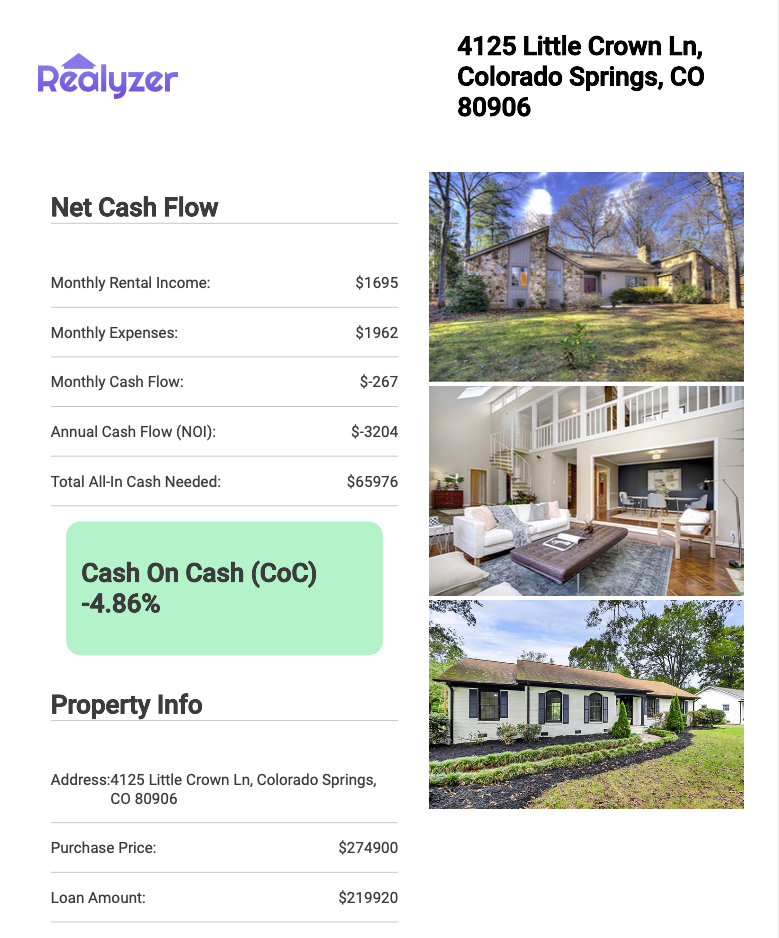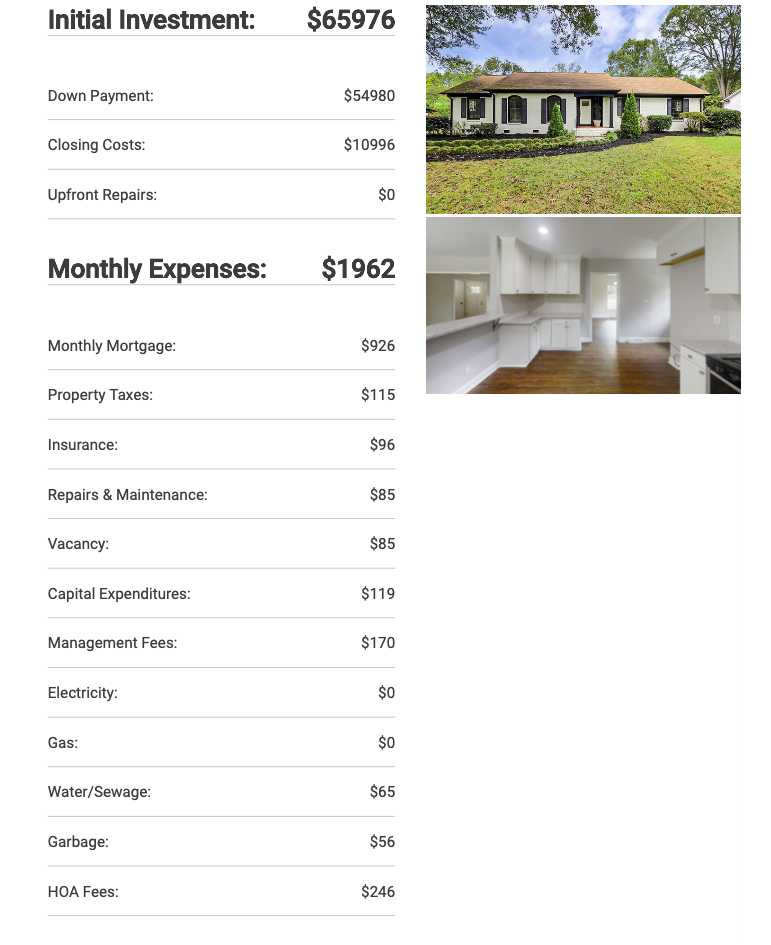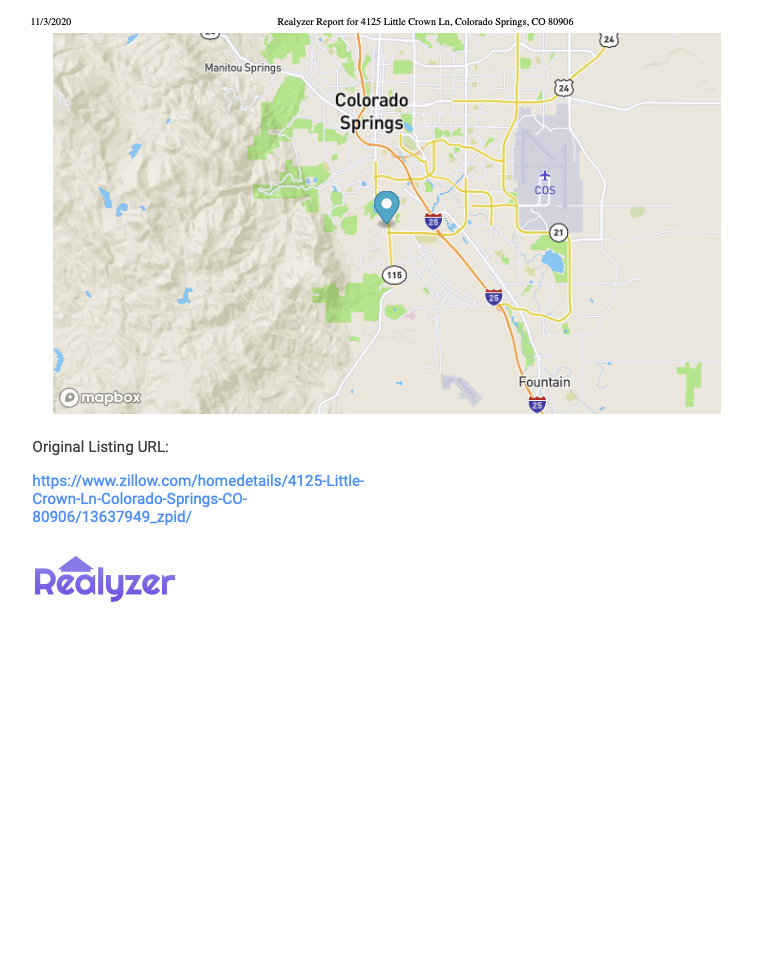# How To Use

Realyzer was created for any level real estate investor, from the newbie investor to the experienced. This tool provides a simple and intuitive interface to input your data for any rental property.

Click here for a video demonstration of the app

# Visual Walkthrough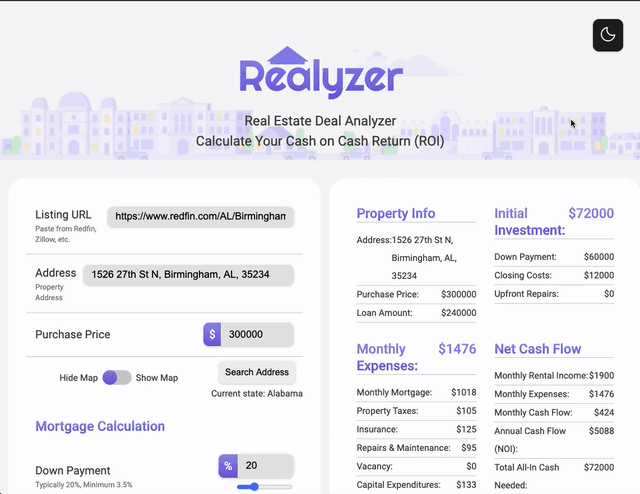# Enter Property Address for Map and Property Tax Estimates: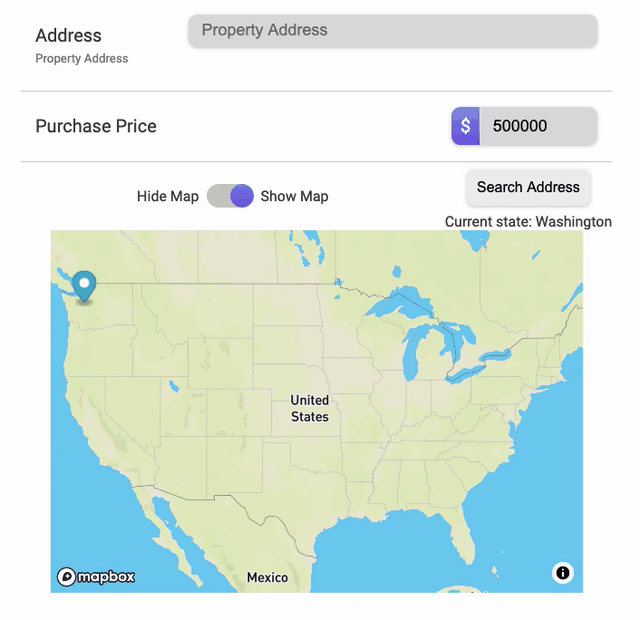# Mortgage Calculation Formula

For the mortgage calculation, the key variables to keep track of were the Property Value, the user's Down Payment Percentage (e.g. 20% down), the Interest Rate (e.g. 4%), and the Length of the Loan (e.g. 30 years). These numbers were then plugged into a complex mortgage calculation formula to determine the user's monthly mortgage payment. The user then has the freedom to adjust the Down Payment and Interest Rate via range inputs to dynamically adjust the monthly payments.

``````  Mortgage calculation formula:
M = P [ r(1 + r)^n ] / [ (1 + r)^n – 1]
P = The Principal loan amount
r = Monthly Interest Rate
n = Number of months required to repay the loan
``````